This notebook is part of the kikuchipy documentation https://kikuchipy.org. Links to the documentation won't work from the notebook.

# M&M 2021 Sunday Short Course¶

Note This notebook was used to demonstrate EBSD analysis with kikuchipy as part of the HyperSpy workshop during the Microscopy & Microanalysis Virtual Meeting in 2021. The offical name of the workshop is [M&M 2021 Sunday Short Course *X-15 Data Analysis in Materials Science*](https://www.microscopy.org/MandM/2021/program/short_courses.cfm). This notebook has been updated to work with the current release of kikuchipy and to fit our documentation format. The original notebook, as presented during the workshop, is available via [this GitHub repository](https://github.com/usnistgov/hyperspy_tutorial/blob/2021-08-01_MandM_short_course/09%20EBSD/ebsd_analysis_nickel_completed.ipynb) hosted by the National Institute of Standards and Technology (NIST).

## Electron Backscatter Diffraction (EBSD) analysis of polycrystalline Nickel¶

The goal of EBSD analysis is often to determine the crystal orientation from each EBSD pattern, typically called indexing. One approach is dictionary indexing, first described in Chen et al. (2015). Here we'll demonstrate how to do this in kikuchipy. The implementation is based on the one in EMsoft, as described in Jackson et al. (2019).

Dictionary indexing is not as tried and tested as the commonly used Hough indexing. To aid the evaluation of dictionary indexing results, we therefore first obtain several maps to get an overview of the quality of the EBSD patterns and the features in the region of interest before indexing, independent of any bias introdued in indexing. After indexing, we'll also inspect the results visually using dynamical and geometrical EBSD simulations.

Set Matplotlib plotting backend and import packages

In :
# Exchange inline for notebook or qt5 (from pyqt) for interactive plotting
%matplotlib inline

import hyperspy.api as hs
import matplotlib.pyplot as plt

# pyxem family of packages (except pyxem)
from diffsims.crystallography import ReciprocalLatticePoint
import kikuchipy as kp
from orix import io, quaternion, sampling, vector

plt.rcParams.update({"figure.facecolor": "w", "font.size": 15})


Load (and download) an EBSD dataset of polycrystalline, recrystallized Nickel which is part of the kikuchipy.data module ("large" = 13 MB, compared to "small" < 1 MB).

In :
s = kp.data.nickel_ebsd_large(allow_download=True)
s

Out:
<EBSD, title: patterns Scan 1, dimensions: (75, 55|60, 60)>

Inspect the navigation and signal dimensions (more closely) in the axes_manager

In :
print(s.axes_manager)

<Axes manager, axes: (75, 55|60, 60)>
Name |   size |  index |  offset |   scale |  units
================ | ====== | ====== | ======= | ======= | ======
x |     75 |      0 |       0 |     1.5 |     um
y |     55 |      0 |       0 |     1.5 |     um
---------------- | ------ | ------ | ------- | ------- | ------
dx |     60 |        |       0 |       1 |     um
dy |     60 |        |       0 |       1 |     um


Plot the data (by navigating the patterns in a mean intensity map) with plot()

In :
s.plot()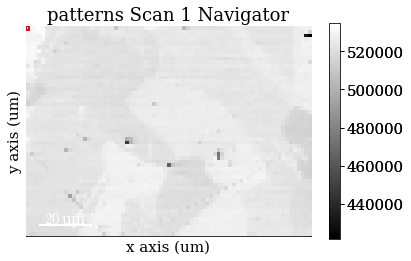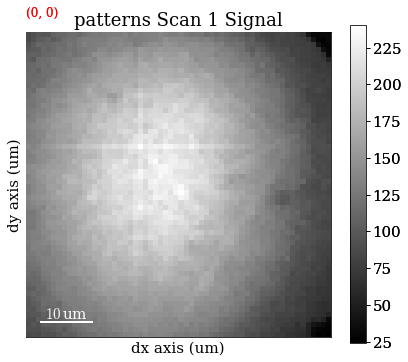Note that kikuchipy has a kikuchipy.load() function almost identical to hyperspy.api.load(), which can read several commercial EBSD formats. See the IO user guide for more information.

## Pre-pattern-processing maps¶

### Mean intensity in each pattern¶

Get the map of the mean intensity in each pattern with mean()

In :
mean_intensity = s.mean(axis=(2, 3))
mean_intensity

Out:
<BaseSignal, title: patterns Scan 1, dimensions: (75, 55|)>

Plot the mean intensity map

In :
mean_intensity.plot()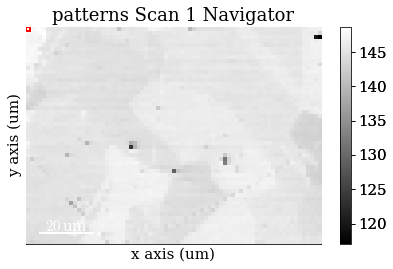### Virtual backscatter electron (VBSE) images¶

Inspect angle resolved backscatter electron (BSE) images, typically called VBSE/vBSE/virtual diode imaging.

Create a VirtualBSEGenerator

In :
vbse_gen = kp.generators.VirtualBSEGenerator(s)
vbse_gen

Out:
VirtualBSEGenerator for <EBSD, title: patterns Scan 1, dimensions: (75, 55|60, 60)>

#### One image per VBSE grid tile¶

Separate the EBSD detector (signal dimensions) into a (3 x 3) grid by setting grid_shape

In :
vbse_gen.grid_shape = (3, 3)


Plot the grid with plot_grid()

In :
vbse_gen.plot_grid();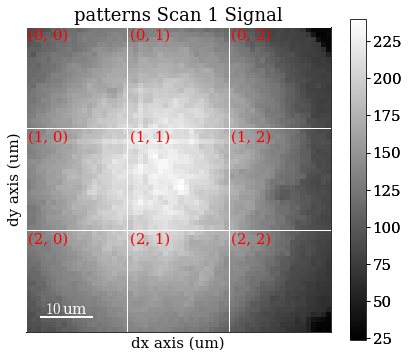Get one VBSE image from the intensity within each grid tile with get_images_from_grid()

In :
vbse_imgs = vbse_gen.get_images_from_grid()
vbse_imgs

Out:
<VirtualBSEImage, title: , dimensions: (3, 3|75, 55)>

Plot the images (one by one)

In :
vbse_imgs.plot()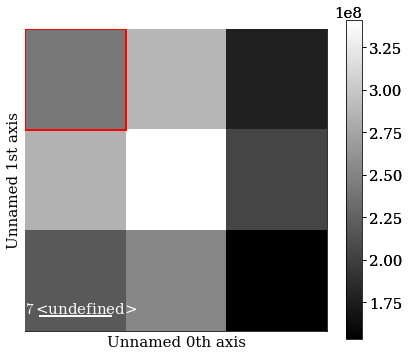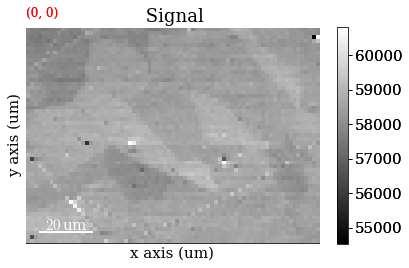Strech the image contrast in each VBSE image by setting the darkest intensities to 0 and the highest intensities to 255 within the 0.5% percentiles, using rescale_intensity()

In :
vbse_imgs.rescale_intensity(percentiles=(0.5, 99.5))

Rescaling the image intensities:
[########################################] | 100% Completed |  0.2s


Replot the images after intensity rescaling in a nice image grid using HyperSpy's plot_images()

In :
fig = plt.figure(figsize=(10, 10))
hs.plot.plot_images(vbse_imgs, fig=fig);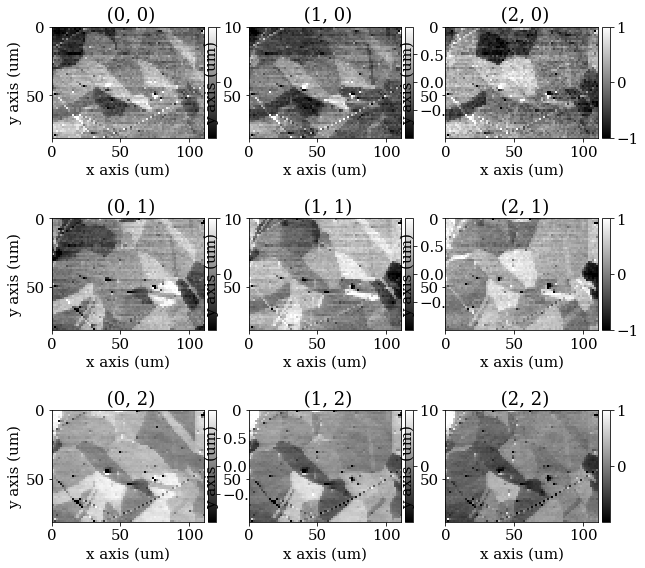#### RGB image¶

Separate the EBSD detector into a (5 x 5) grid

In :
vbse_gen.grid_shape = (5, 5)


Set some (can be more than one) of the grid tiles to be coloured red, green, or blue, and plot the color key

In :
rgb = [(2, 1), (2, 2), (2, 3)]
vbse_gen.plot_grid(rgb_channels=rgb)

Out:
<EBSD, title: patterns Scan 1, dimensions: (|60, 60)>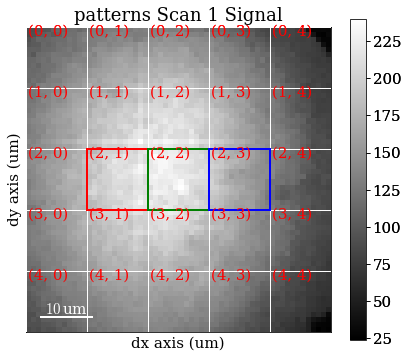Create an RGB image from the specified grid tiles with get_rgb_image()

In :
vbse_rgb_img = vbse_gen.get_rgb_image(*rgb)
vbse_rgb_img

Out:
<VirtualBSEImage, title: , dimensions: (|75, 55)>

Plot the resulting image

In :
vbse_rgb_img.plot()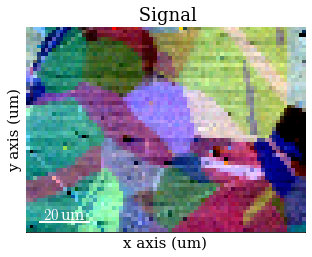## Process pattern intensities¶

The raw EBSD signal can be empirically evaluated as a superposition of a Kikuchi diffraction pattern and a smooth background intensity. For pattern indexing, the latter intensity is usually undesirable, while for VBSE imaging, as we saw above, this intensity can reveal topographical, compositional or diffraction contrast.

### Remove the static background¶

Effects which are constant, like hot pixels or dirt on the detector, can be removed by either subtracting or dividing by a static background. Ideally, this background pattern has no signal of interest.

A static background pattern was acquired with the Nickel EBSD data set, which was loaded with the data set into the signal metadata.

Retrieve this background from the metadata and plot it

In :
bg = s.metadata.Acquisition_instrument.SEM.Detector.EBSD.static_background

fig, ax = plt.subplots()
ax.imshow(bg, cmap="gray");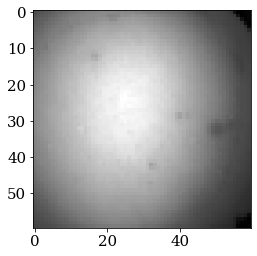If one is not available, we can try to generate a suitable static background by averaging all patterns (and reverting the data type to 8-bit unsigned integers)

In :
bg2 = s.mean(axis=(0, 1))
bg2.change_dtype(s.data.dtype)


Compare it to the background from the metadata by plotting it

In :
bg2.plot()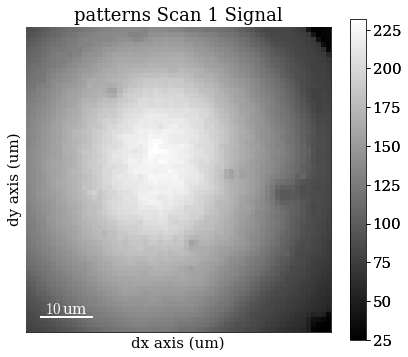Remove the static background with remove_static_background()

In :
s.remove_static_background()

Removing the static background:
[########################################] | 100% Completed |  0.2s


### Remove the dynamic background¶

Uneven intensity in a static background subtracted pattern can be corrected by subtracting or dividing by a dynamic background obtained by Gaussian blurring. A Gaussian window with a standard deviation set by std is used to blur each pattern individually (dynamic) either in the spatial or frequency domain. Blurring in the frequency domain is effectively accomplished by a low-pass Fast Fourier Transform (FFT) filter. The individual Gaussian blurred dynamic backgrounds are then subtracted or divided from the respective patterns.

Remove the dynamic background with remove_dynamic_background()

In :
s.remove_dynamic_background()

Removing the dynamic background:
[########################################] | 100% Completed |  1.0s


### Average neighbour patterns¶

The signal-to-noise ratio in patterns can be improved by averaging patterns with their closest neighbours within a window/kernel/mask.

Let's average with all eight nearest neighbours, but use Gaussian weights with a standard deviation of 1. Create the Gaussian filters.Window and plot it

In :
w = kp.filters.Window(window="gaussian", std=1)
w.plot()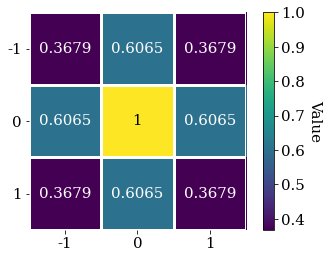Average all patterns with their neighbour patterns using the Gaussian window with average_neighbour_patterns()

In :
s.average_neighbour_patterns(window=w)

Averaging with the neighbour patterns:
[########################################] | 100% Completed |  0.5s


We can subsequently save these patterns to kikuchipy's own h5ebsd specification Jackson et al. (2014) for the general format). This format can be read back into kikuchipy, or as a file in the EMEBSD format in the powerful suite of EMsoft command line programs.

In :
#s.save("pattern_static_dynamic_averaged.h5")


Note that neighbour pattern averaging increases the virtual interaction volume of the electron beam with the sample, leading to a potential loss in spatial resolution. Averaging may in some cases, like on grain boundaries, mix two or more different diffraction patterns, which might be unwanted. See Wright et al. (2015) for a discussion of this concern.

## Pre-indexing maps¶

The image quality metric Q presented by Krieger Lassen (1994) relies on the assumption that the sharper the Kikuchi bands, the greater the high frequency content of the FFT power spectrum, and thus the closer Q will be to unity. It can from this be expected that grain interiors will show a high Q, while grain boundaries will show a lower Q.

Get the image quality map with get_image_quality()

In :
iq = s.get_image_quality()

Calculating the image quality:
[########################################] | 100% Completed |  1.0s


We can also produce a map showing how similar each pattern is to their four nearest neighbour (or any other number of neighbours specified by a binary mask). Get the average neighbour dot product map with get_average_neighbour_dot_product_map()

In :
adp = s.get_average_neighbour_dot_product_map()

Calculating average neighbour dot product map:
[########################################] | 100% Completed |  1.1s


Let's plot them side by side with colorbars using Matplotlib

In :
fig, ax = plt.subplots(ncols=2, figsize=(9, 3))

im0 = ax.imshow(iq)
ax.axis("off")
fig.colorbar(im0, ax=ax, label="Image quality")

ax.axis("off")
fig.colorbar(im1, ax=ax, label="Ave. neighbour dot product")

fig.tight_layout();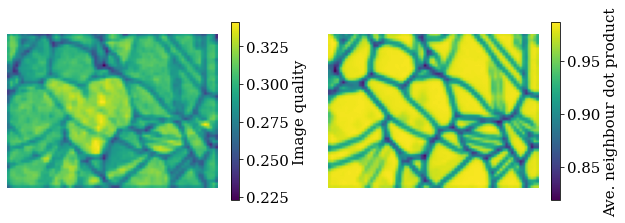## Dictionary indexing¶

Now we're ready to set up and run dictionary indexing of the background corrected and averaged patterns.

Before we can generate a dictionary of simulated patterns, we need a dynamically simulated master pattern containing all possible scattering vectors for a candidate phase. This can be simulated using EMsoft (Callahan and De Graef ) and subsequently imported into kikuchipy using kikuchipy.load().

For demonstration purposes, we've included small (401 x 401) master patterns of Nickel in the stereographic and Lambert (square) projections as part of the kikuchipy.data module. Load the 20 keV master pattern from the northern hemisphere in the Lambert projection

In :
mp = kp.data.nickel_ebsd_master_pattern_small(projection="lambert", energy=20)
mp

Out:
<EBSDMasterPattern, title: ni_mc_mp_20kv_uint8_gzip_opts9, dimensions: (|401, 401)>

Plot the master pattern

In :
mp.plot()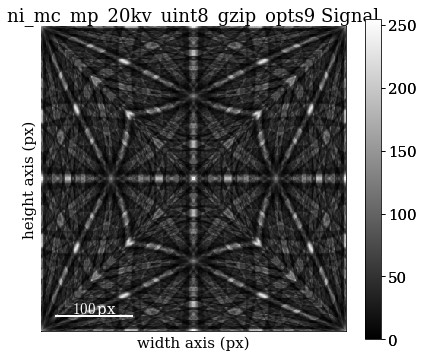Extract phase information loaded with the master pattern

In :
phase = mp.phase
phase

Out:
<name: ni. space group: Fm-3m. point group: m-3m. proper point group: 432. color: tab:blue>

Inspect it's crystal structure (list of asymmetric atom positions and a structure.lattice)

In :
phase.structure

Out:
[28   0.000000 0.000000 0.000000 1.0000]
In :
phase.structure.lattice

Out:
Lattice(a=0.35236, b=0.35236, c=0.35236, alpha=90, beta=90, gamma=90)

### Sample orientation space¶

Here we produce a sampling of the Rodriguez Fundamental Zone (RFZ) of point group $m\bar{3}m$ using a "characteristic distance" or "resolution" of 4$^{\circ}$, as implemented in orix. This resolution is quite coarse, and used here because of time and memory constraints. We, and EMsoft (see the aforementioned tutorial article by Jackson et al.), suggest using a smaller resolution of about 1.5$^{\circ}$ for experimental work.

Sample the RFZ for the Ni phase space group with a resolution of 4$^{\circ}$ using orix.sampling.get_sample_fundamental() and inspect the results

In :
rot = sampling.get_sample_fundamental(
method="cubochoric", resolution=4, point_group=phase.point_group
)
rot

Out:
Rotation (11935,)
[[ 0.8606 -0.3337 -0.3337 -0.1912]
[ 0.8606 -0.3397 -0.3397 -0.1687]
[ 0.8606 -0.345  -0.345  -0.1456]
...
[ 0.8606  0.345   0.345   0.1456]
[ 0.8606  0.3397  0.3397  0.1687]
[ 0.8606  0.3337  0.3337  0.1912]]

### Define the sample-detector geometry¶

Now that we have our master pattern and crystal orientations, we need to describe the EBSD detector’s position with respect to the sample. This ensures that projecting parts of the master pattern onto our detector yields dynamically simulated patterns presumably resembling our experimental ones. The average projection/pattern center (PC) for this experiment was determined by indexing five calibration patterns using the EDAX TSL Data Collection v7 software, and is ($x^*$, $y^*$, $z^*$) = (0.4210, 0.7794, 0.5049).

Create the detector.EBSDDetector and inspect it

In :
detector = kp.detectors.EBSDDetector(
shape=s.axes_manager.signal_shape[::-1],
sample_tilt=70,
pc=(0.421, 0.7794, 0.5049),
convention="tsl"
)
detector

Out:
EBSDDetector (60, 60), px_size 1 um, binning 1, tilt 0, azimuthal 0, pc (0.421, 0.221, 0.505)

Let's double check the projection/pattern center (PC) position on the detector by plotting it in gnomonic coordinates and showing the gnomonic circles at 10$^{\circ}$ steps

In :
detector.plot(coordinates="gnomonic", pattern=s.inav[0, 0].data, draw_gnomonic_circles=True)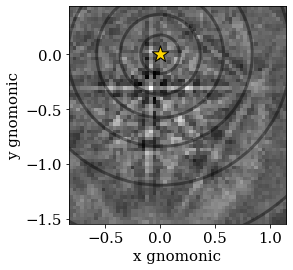### Generate dictionary of simulated patterns¶

Now we’re ready to generate our dictionary of simulated patterns by projecting parts of the master pattern onto our detector for all sampled orientations. The method assumes the crystal orientations are represented with respect to the EDAX TSL sample reference frame RD-TD-ND. For more details, see the reference frame user guide.

So, generate a dictionary of simulated patterns using MasterPattern.get_patterns()

In :
dynsim = mp.get_patterns(
rotations=rot,
detector=detector,
energy=20,
dtype_out=s.data.dtype,
compute=True
)
dynsim

Creating a dictionary of (11935,) simulated patterns:
[########################################] | 100% Completed |  2.3s

Out:
<EBSD, title: , dimensions: (11935|60, 60)>

We've now generated the dictionary and read it into memory. We could instead have passed compute=False, which would have returned a LazyEBSD to be computed during the indexing run. This can sometimes be desirable.

Let's inspect a few of the simulated patterns to ensure they look alright by plotting them

In :
dynsim.plot()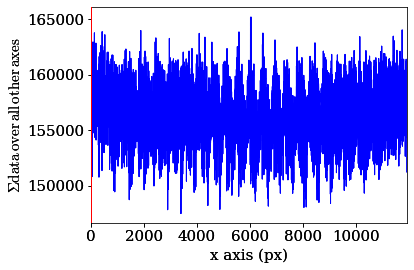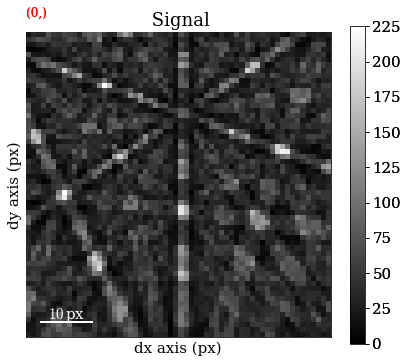### Perform dictionary indexing¶

Finally, let's match the simulated patterns to our experimental patterns, using the zero-mean normalized cross correlation (NCC) coefficient, which is the default similarity metric. We'll keep the 10 best matching orientations. A number of about 4125 * 12000 comparisons is quite small, which we can do in memory all at once. However, in cases where the number of comparisons are too big for our memory to handle, we can iterate over the dictionary to match only parts at a time. We'll use at least 20 iterations here.

Let's perform dictionary_indexing()

In :
xmap = s.dictionary_indexing(
dictionary=dynsim,
metric="ncc",
keep_n=10,
)

Dictionary indexing information:
Phase name: ni
Matching 4125 experimental pattern(s) to 11935 dictionary pattern(s)
NormalizedCrossCorrelationMetric: float32, greater is better, rechunk: False, signal mask: False

100%|████████████████████████████████████████████████████████████████████| 21/21 [00:05<00:00,  3.82it/s]


Inspect the returned CrystalMap

In :
xmap

Out:
Phase   Orientations  Name  Space group  Point group  Proper point group     Color
0  4125 (100.0%)    ni        Fm-3m         m-3m                 432  tab:blue
Properties: scores, simulation_indices
Scan unit: px

We can write the indexing results to file using one of orix' writers. orix' own HDF5 file format stores all results to in HDF5 file, while the .ang file writer only stores the best matching orientation

In :
#io.save("di_results_ni1.h5", xmap)
#io.save("di_results_ni1.ang", xmap)


## Inspect indexing results¶

### Indexing maps¶

So far, orix cannot produce any orientation color maps, pole figures etc. This has to be done in other software, like MTEX.

Here, we'll inspect the map of best matching scores and a so-called orientation similarity map, which compares the best matching orientations for each pattern to it's nearest neighbours.

Get the NCC map in the correct shape from the CrystalMap's scores property

In :
ncc_map = xmap.scores[:, 0].reshape(*xmap.shape)


Get the indexing.orientation_similarity_map() using the full list of 10 best matches per pattern/point

In :
os_map = kp.indexing.orientation_similarity_map(xmap)


Plot the maps using Matplotlib

In :
fig, ax = plt.subplots(ncols=2, figsize=(9, 3))

im0 = ax.imshow(ncc_map)
ax.axis("off")
fig.colorbar(im0, ax=ax, label="NCC")

im1 = ax.imshow(os_map)
ax.axis("off")
fig.colorbar(im1, ax=ax, label="Orientation similarity")

fig.tight_layout();